Next: Poisson model Up: Inferring numerical values of Previous: Gaussian model

## Binomial model

In a large class of experiments, the observations consist of counts, that is, a number of things (events, occurrences, etc.). In many processes of physics interests the resulting number of counts is described probabilistically by a binomial or a Poisson model. For example, we want to draw an inference about the efficiency of a detector, a branching ratio in a particle decay or a rate from a measured number of counts in a given interval of time.

The binomial distribution describes the probability of randomly obtaining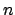events (successes') in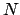independent trials, in each of which we assume the same probabilitythat the event will happen. The probability function is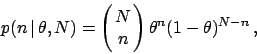(37)

where the leading factor is the well-known binomial coefficient, namely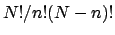. We wish to inferfrom an observed number of countsintrials. Incidentally, that was the `problem in the doctrine of chances'' originally treated by Bayes (1763), reproduced e.g. in (Press 1992). Assuming a uniform prior for, by Bayes' theorem the posterior distribution foris proportional to the likelihood, given by Eq. (37):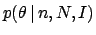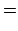(38)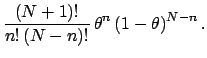(39)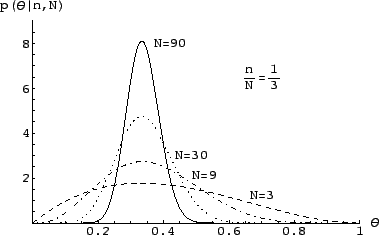Some examples of this distribution for various values ofandare shown in Fig. 1. Expectation, variance, and mode of this distribution are: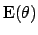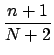(40)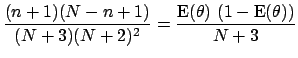(41)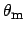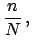(42)

where the mode has been indicated with. Equation (40) is known as the Laplace formula. For large values ofand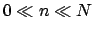the expectation oftends to, andbecomes approximately Gaussian. This result is nothing but a reflection of the well-known asymptotic Gaussian behavior of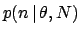. For largethe uncertainty aboutgoes like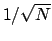. Asymptotically, we are practically certain thatis equal to the relative frequency of that class of events observed in the past. This is how the frequency based evaluation of probability is promptly recovered in the Bayesian approach, under well defined assumptions.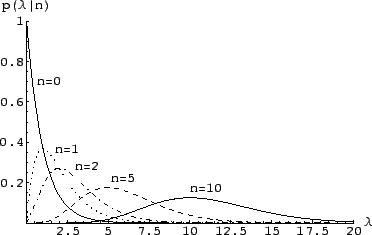Next: Poisson model Up: Inferring numerical values of Previous: Gaussian model
Giulio D'Agostini 2003-05-13